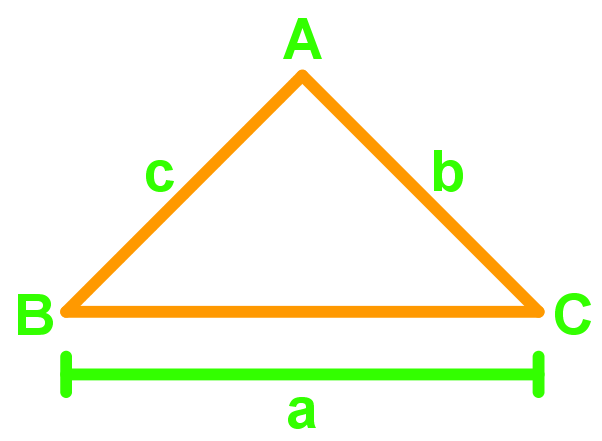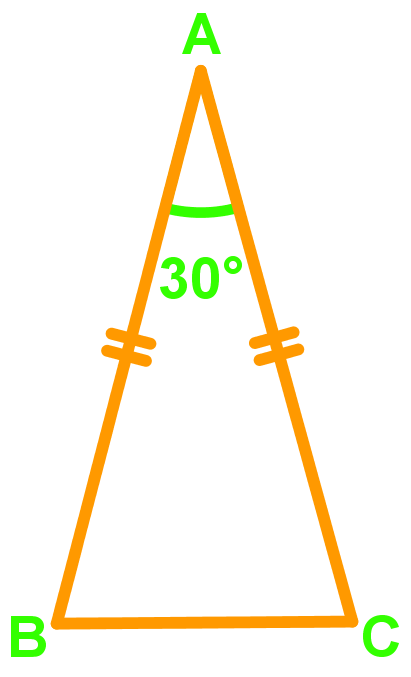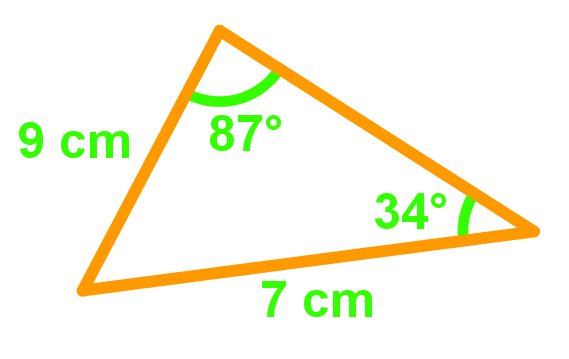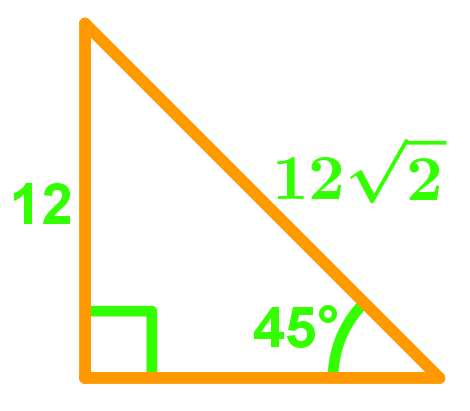# Area of triangles: 1/2 a*b sin(C)#### Everything You Need in One Place

Homework problems? Exam preparation? Trying to grasp a concept or just brushing up the basics? Our extensive help & practice library have got you covered.#### Learn and Practice With Ease

Our proven video lessons ease you through problems quickly, and you get tonnes of friendly practice on questions that trip students up on tests and finals.#### Instant and Unlimited Help

Our personalized learning platform enables you to instantly find the exact walkthrough to your specific type of question. Activate unlimited help now!

##### Intros
###### Lessons
1. Introduction to Area of Triangles: $\frac{1}{2} ab\sin C$
##### Examples
###### Lessons
1. Proof: Area of Triangle = $\frac{1}{2} ab\sin C$

Using the given diagram, prove that the area of a triangle can be found by the equation $\frac{1}{2} ab\sin C$1. Finding the Area of a Triangle Given 2 Sides and the Angle in Between

$\Delta XYZ$ has side lengths $XY$=9cm, $XZ$=12cm and $\angle YXZ$=42°. Find the area of $\Delta XYZ$. Give your answer to 1 decimal place.

1. Area of Isosceles Triangles

The area of an isosceles triangle $\Delta ABC$ is 42.25 $cm^{2}$. If $\angle A$=30°, find the length of $AB$ in exact value.1. Area of Equilateral Triangles

An equilateral triangle has side length 4cm. Find its area and give your answer in exact value.

1. Determining the Areas of Different Triangles

Find the area of the following triangle.1. Find the area of the following right-angled triangle.###### Topic Notes
In this lesson, we will learn:
• Proof: Area of Triangle =$\frac{1}{2}ab \sin C$
• Finding the Area of a Triangle Given 2 Sides and the Angle in Between
• Area of Isosceles Triangles
• Area of Equilateral Triangles
• Determining the Areas of Different Triangles

Notes:
• The base and height of a triangle must be perpendicular to each other.
• The traditional formula for the area of a triangle = $\frac{1}{2} \times base \times height$
• An isosceles triangle has two sides of equal length.
• An equilateral triangle has three sides of equal length and all the inner angles equal to 60°.
• Angles inside a triangle add up to 180°.
• Pythagorean Theorem: $a^{2}+b^{2}=c^{2}$